Almost Matching Exactly

For Interpretable Treatment Effect Estimation

 Marco Morucci Duke University

marco.morucci@duke.edu

## Treatment Effects and High-Stakes Decisions

Causal Estimates are increasingly used to justify decisions in all sectors...

 PoliticsBusinessHealthcareGoal: We want to provide decision-makers with trustworthy causal estimates that produce justifiable estimates.

### Treatment effect estimation

#### Setting: Observational Causal Inference

• We have a set of $N$ units
• They have potential outcomes $Y_i(1), Y_i(0)$
• They are associated with a set of contextual covariates, $\mathbf{X}_i$
• They are assigned either treatment $T_i=1$ or $T_i=0$
• We observe $Y_i = T_iY(1) + (1-T_i)Y_i(0)$
• We would like to know: $$CATE(\mathbf{x}_i) = \mathbb{E}[Y_i(1) - Y_i(0)|\mathbf{X_i = \mathbf{x}_i}]$$
• Assume SUTVA, Overlap, ignorability

All methods presented here can also be applied to experimental data, for example, to analyze treatment effect heterogeneity.

### Treatment effect estimation

How do we estimate a quantity that's half unobserved?

We can under two main assumptions

• Conditional Ignorability $(Y_i(1), Y_i(0))$ is independent of $T_i$ conditional on $\mathbf{X}_i$
• SUTVA: Unit $i$ is exclusively affected by its own treatment

### Matching in a nutshell

Matching allows us to take advantage of CI and SUTVA to estimate the CATE in an interpretable way.

• For each unit, $i$ find several other units that are similar in the covariate values.
• Form a Matched Group (MG) with all the units matched to $i$
• Estimate the CATE for $i$ with $$\widehat{CATE}_i = \frac{1}{N^t_{MG}}\sum_{i \in MG}Y_iT_i - \frac{1}{N^c_{MG}}\sum_{i \in MG}Y_i(1-T_i)$$

### Why matching?

We choose matching to estimate CATEs because matching is interpretable and interpretability is important

• Interpretability permits greater accountability of decision-makers in high-stake settings
• Interpretability allows researchers to debug their models, datasets and results
• Interpretability makes results from Machine Learning tools more trustworthy to human observers

### Matching is interpretable because estimates are case-based

"We came up with that estimates because we looked at these similar cases."

### But is case choice interpretable in existing methods?

Case-based estimation is good, but can we do better?

• Most popular matching methods match on an uniterpretable single-number summary (propensity, prognostic score) of the covariates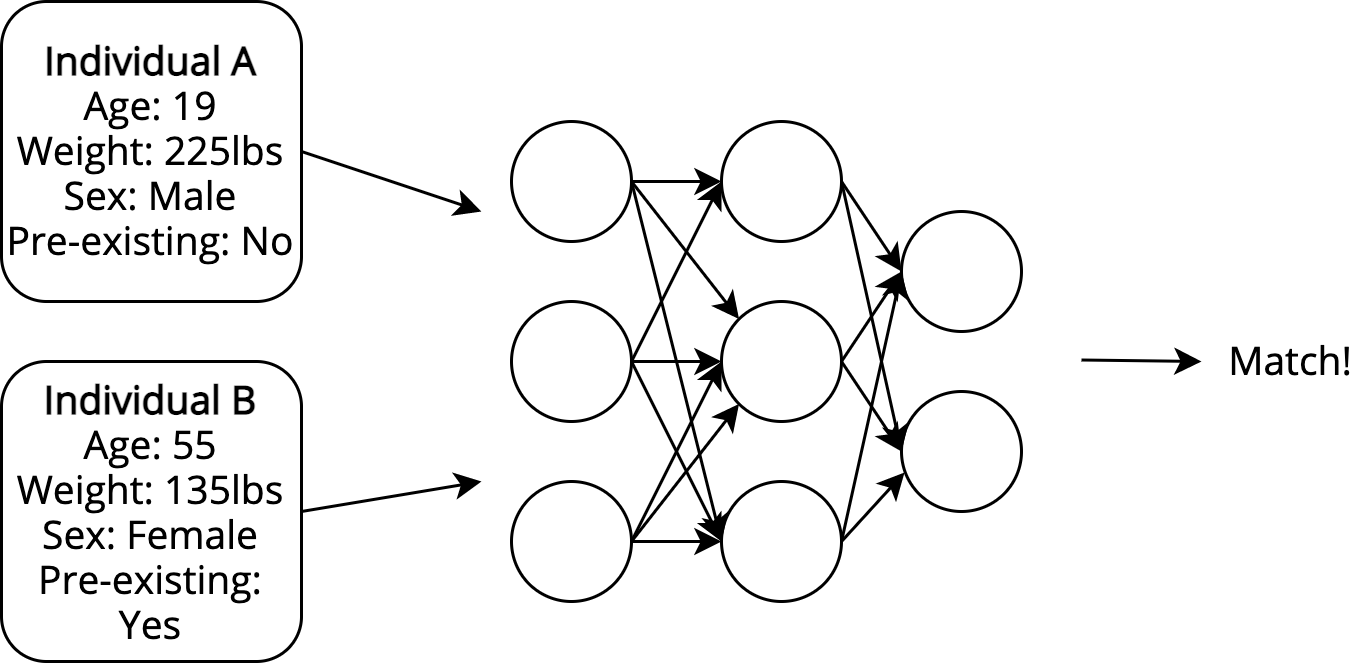• Case choice is unexplainable!
• We want to be able to explain why two units were matched together

### But do existing matching methods produce accurate CATE estimates?

Matching estimates can be inaccurate

• Complex and flexible ML methods are likely to make better predictions than simple matches
• Can we bridge the gap between methods?

The rest of this talk will cover

## Almost Matching Exactly

A family of matching methods that:

• Make explainable matched groups
• Rely on flexible ML to make accurate predictions
• Are fast in most applied settings
• Can be scaled up to massive datasets in some cases

### Two Algorithms

• FLAME for scalable matching on discrete data
• AHB for accurate matching on continuous data

### Almost Matching Exactly With Discrete Covariates

Given a unit, choose a set of units with the opposite treatments to match it to.

Ideally, we would match it to units with exactly the same covariates

Problem: There are no exact matches on all covariates!

Solution: Make an Almost-Exact Match on the most important covariates.

### Almost Matching Exactly With Discrete Covariates

Step 1 Split your data into a training set and a matching set, the latter containing the unit you wish to match.### Almost Matching Exactly With Discrete Covariates

Step 2 Using Machine Learning on the training set, figure out which covariates predict the outcome best### Almost Matching Exactly With Discrete Covariates

Step 2 Using Machine Learning on the training set, figure out which covariates predict the outcome best### Almost Matching Exactly With Discrete Covariates

Step 3 Find the set of covariates that maximizes cumulative weight, and permits an exact match with at least one other unit.Age Height Degree Nothing 15 10 5 Age -- 25 20 Height 25 -- 15 Degree 20 15 --

We should match on Age and Height!

### Almost Matching Exactly With Discrete Covariates

Step 4 Match that unit exactly on that set of covariates with as many other units as possible.That's it!

### Almost Matching Exactly Formally

\begin{align} &\boldsymbol{\theta}_i^* \in \text{arg}\max\limits_{\boldsymbol{\theta} \in \{0,1\}^p} \mathbf{w}^T\boldsymbol{\theta}\\ &\text{s.t.:}\, \exists j:\, T_j\neq T_i,\text{ and, } \mathbf{x}_j \circ \boldsymbol{\theta} = \mathbf{x}_i \circ \boldsymbol{\theta}. \end{align}

• $\boldsymbol{\theta}_i$ = covariate selector
• $\mathbf{w}$ = covariate weights, training set variable accuracy in our case

"Among all the subsets of covariates for which there exists at least one exact match with opposite treatment, choose the one that maximizes total weight."

### AME targets estimation error

The CATE estimation error for AME can be upper-bounded as:

$|\widehat{CATE}(\mathbf{x}_i) - CATE(\mathbf{x}_i)| \leq 2M\mathbf{w}^T(1-\boldsymbol{\theta}_i)$

• $\mathbf{w}^T(1-\boldsymbol{\theta}_i)$ is the opposite of $\mathbf{w}^T\boldsymbol{\theta}_i$
• AME maximizes $\mathbf{w}^T\boldsymbol{\theta}_i$

AME minimizes this upper bound on estimation error.

### Fast Large Scale Almost Matching Exactly (FLAME)

[Wang, Moruccy, Awan, Liu, Roy, Rudin, Volfovsky (JMLR 2021)]

• Solves the AME problem very quickly on massive amounts of data
• Can be implemented natively on SQL databases
• No free lunch: FLAME's solution to AME is approximate
• ...but not too far from the optimum.

### The FLAME Algorithm

1. Estimate covariate weights on training set
2. Make as many exact matches as possible, remove matched units from matching set
3. Remove the lowest-weight covariate
4. Repeat until either:
• No more unmatched units are left
• Combined predictive accuracy of remaining covariates dips below threshold (recommended)

### FLAME's performance: simulated data

• 20K Units, half treatment, half control
• 30 covariates, only 10 matter
• Irrelevant covariates affect treatment assignment but not outcome
• Nonlinear outcome model with interaction term

### FLAME's performance: simulated data

• $N^t = N^c = 10,000$, $P = 30$
• $y = \sum_{i=1}^{10}\alpha_ix_i + T\sum_{i=1}^{10}\beta_ix_i + T\sum_{i=1}^5\sum_{j = i}^5 x_ix_j$
• for $i = 1, \dots, 10$ (relevant covariates)
• $x_i \sim Ber(0.5)$
• $\beta_i \sim N(1.5, 0.15)$
• $s \sim Unif(-1, 1), \alpha_i \sim N(10s, 1)$

• for $i = 11, \dots, 30$ (irrelevant covariates)
• $x_i \sim TBer(0.9) + (1-T)Ber(0.1)$
• $\alpha_i = 0$, $\beta_i = 0$

### Simulation Results FLAME vs. other matching methods

 FLAME True Pscore Match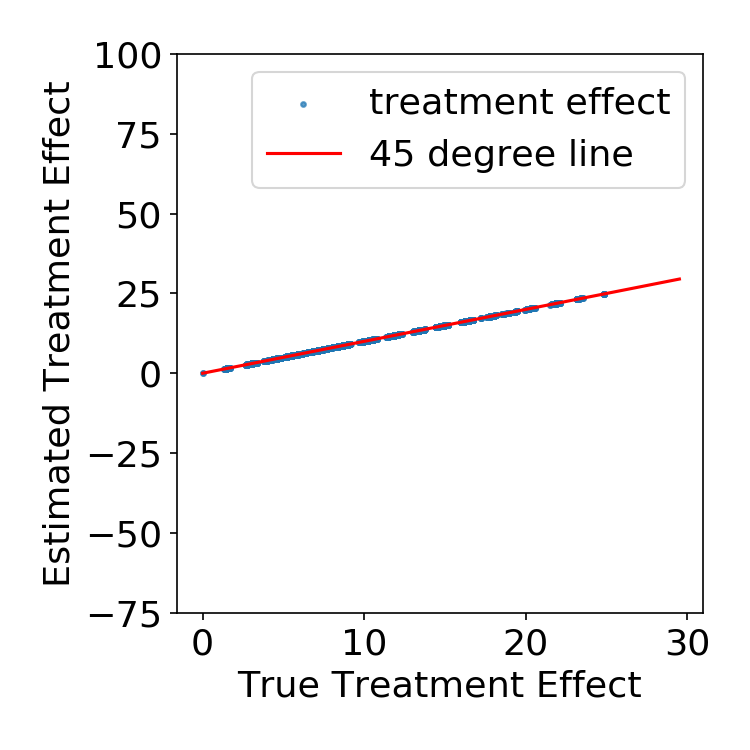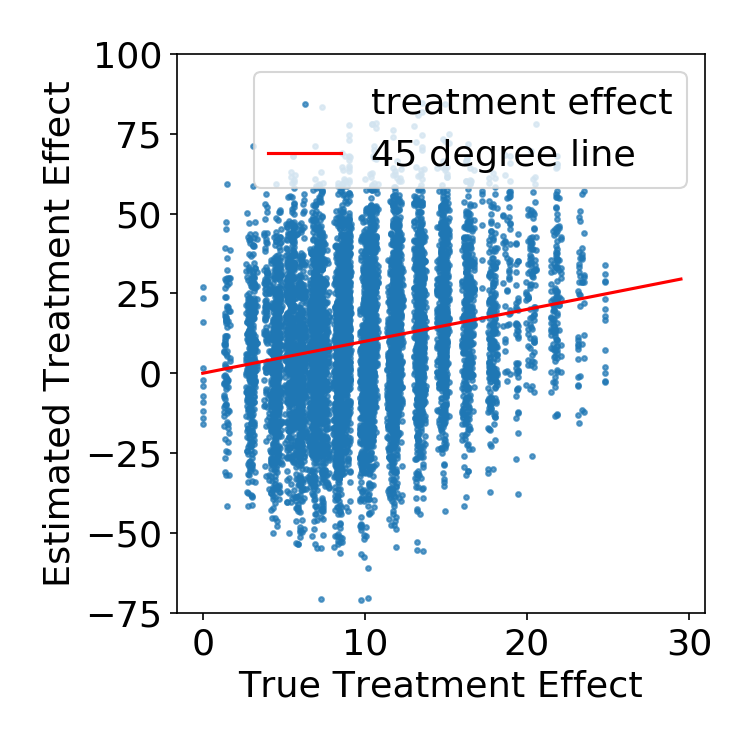### Simulation Results FLAME vs. other matching methods

 FLAME True Pscore Match### Simulation Results FLAME vs. other matching methods

 FLAME Genetic Match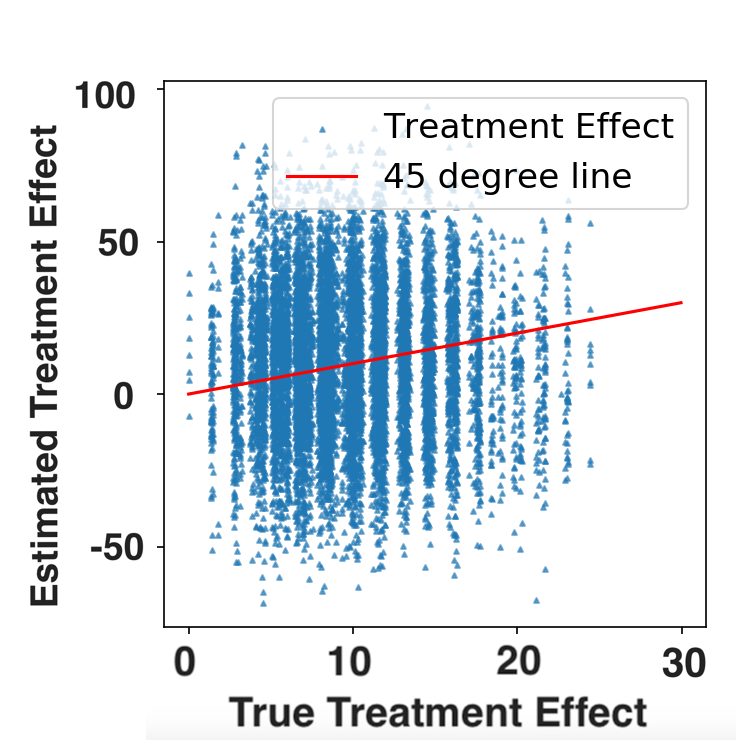### Simulation Results FLAME vs. other matching methods

 FLAME Mahalanobis Match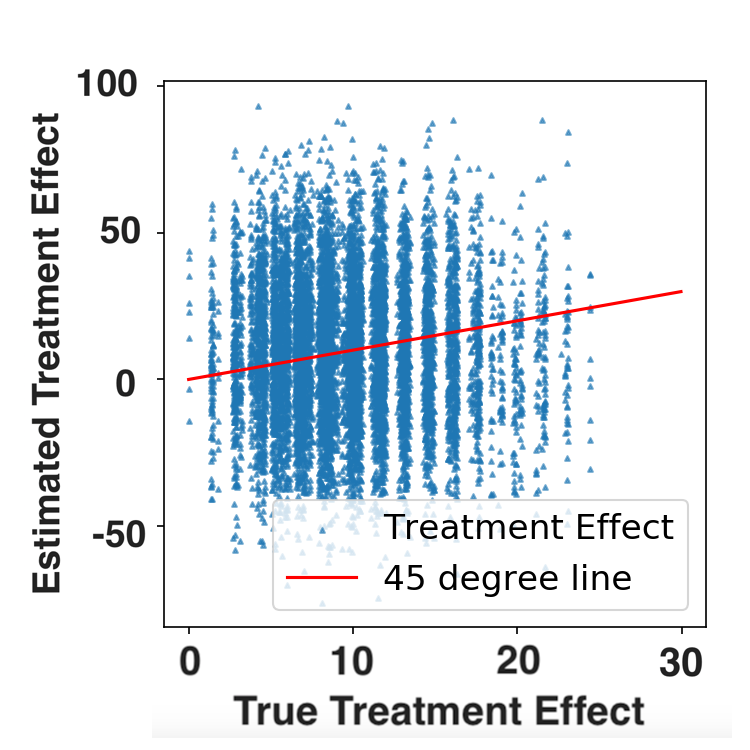### Simulation Results FLAME vs. uninterpretable ML

 FLAME Causal Forests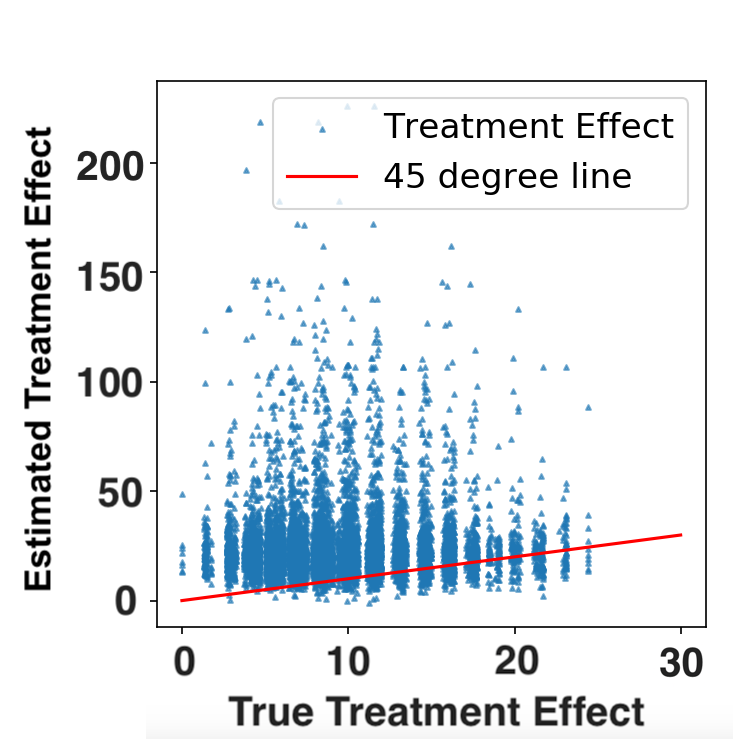### Simulation Results FLAME vs. uninterpretable ML

 FLAME CTMLE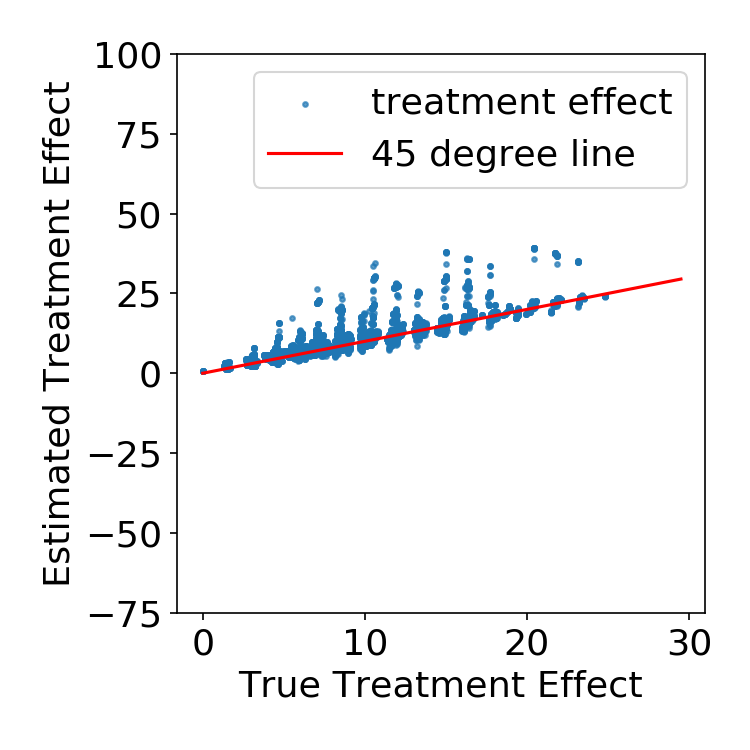### Simulation Results FLAME vs. uninterpretable ML

 FLAME BART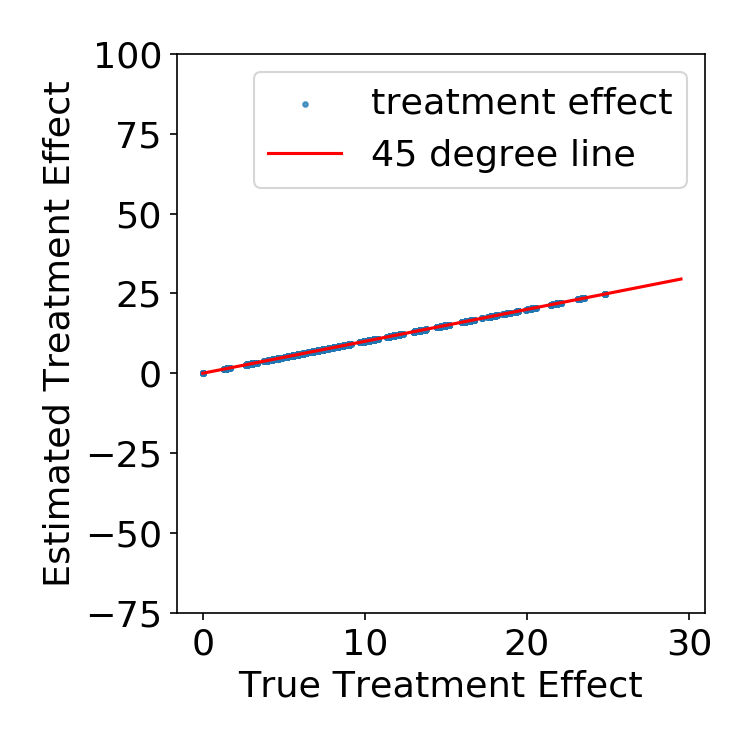### How does FLAME scale?

Method Time for N=20K (sec) Time for N=1.2M (hr)
FLAME 22.30 1.33
Causal Forest 59.68Crashed
PScore Match 13.88> 10
Genmatch > 150> 10
Cardinality Match > 150> 10

### An AME Application: Smoking and Pregnancy

 Data: All US births in 2010 $N \approx 2.1M$ units, $\approx 75K$ treated Treatment: Mother smokes at least 10 cigarettes a day Control: Mother does not smoke Outcome: Birth weight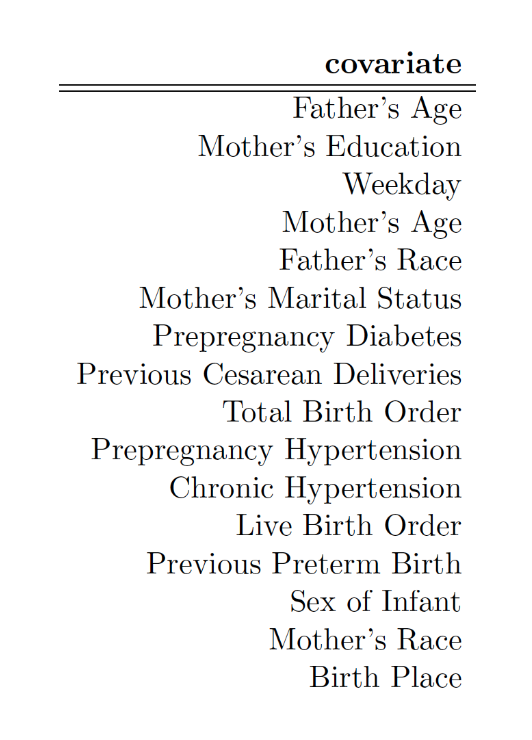### An AME Application: Smoking and Pregnancy

 Data: All US births in 2010 $N \approx 2.1M$ units, $\approx 75K$ treated Treatment: Mother smokes at least 10 cigarettes a day Control: Mother does not smoke Outcome: Birth weight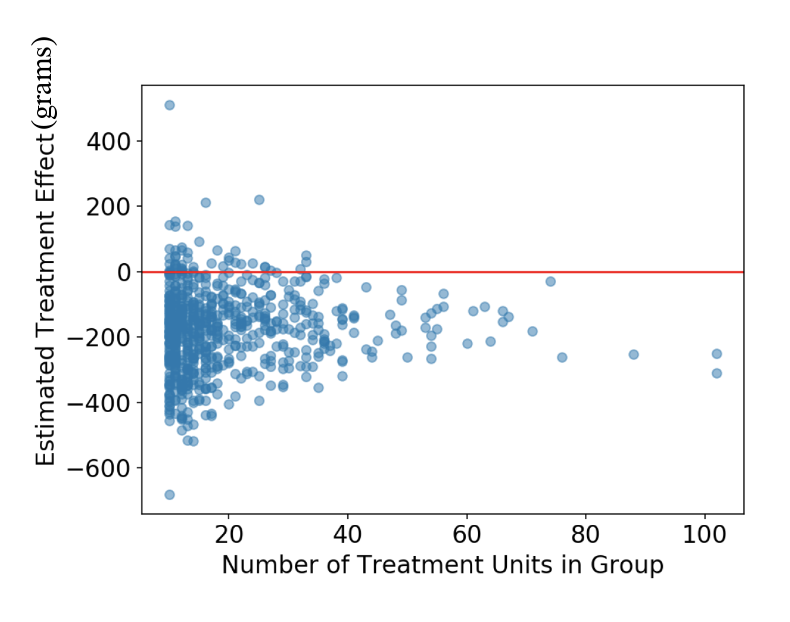### An AME Application: Smoking and Pregnancy

 Data: All US births in 2010 $N \approx 2.1M$ units, $\approx 75K$ treated Treatment: Mother smokes at least 10 cigarettes a day Control: Mother does not smoke Outcome: Birth weight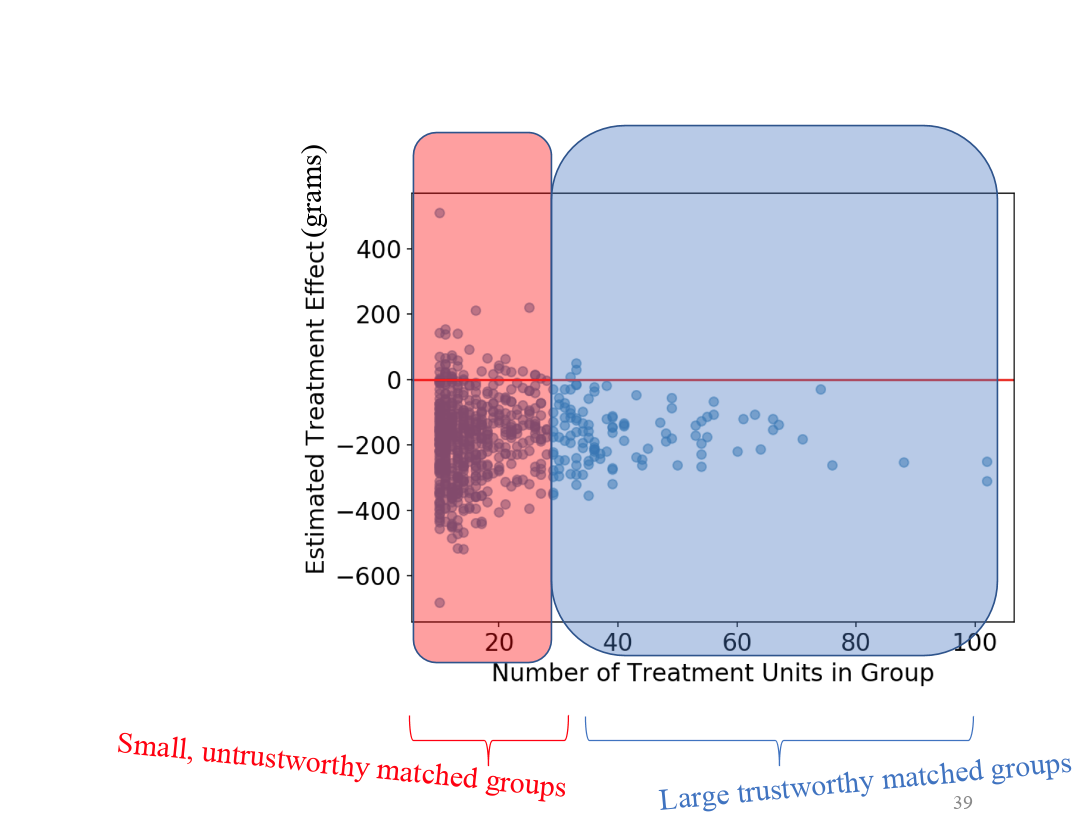### AME widh discrete data and FLAME: summary

• When exact matching on all covariates isn't possible, matching exactly on a subset of covariates is a good solution
• Covariates subsets to match on are chosen with flexible ML tools on a separate training set
• Resulting algorithm is accurate, fast and interpretable

### AME with Continuous Covariates

Problem: do we find almost exact matches when covariates are continuous?

Solution: Coarsening (binning) variables and then using them as inputs for AME seems like a natural solution...

More problems: ...but how do we know how much to coarsen each variable?

### Carefully Coarsening Covariates

Consider the problem of having to bin a covariate, $x$

 Fixed boxes Adaptive boxes### Takeaways

• Relationship between $x$ and $y$ should be considered when binning
• Even so, optimal-but-fixed width bins are not enough, because...
• Different parts of the covariate space should be binned according to variance of $y$

### Takeaways

• Relationship between $x$ and $y$ should be considered when binning
• Even so, optimal-but-fixed width bins are not enough, because...
• Different parts of the covariate space should be binned according to variance of $y$

[Morucci, Orlandi, Roy, Rudin, Volfovsky (UAI 2020)]

An algorithm to adaptively learn optimal coarsenings of the data that satisfy these criteria.

### The AHB Algorithm

Step 1: On a separate training set, fit a flexible ML model to predict both potential outcomes for each unit in the matching set.### The AHB Algorithm

Step 2: Construct a p-dimensional box around each unit, such that, in the box:

 There are many units Predicted outcomes are similar Predictions from outcome averages are similar to ML predictions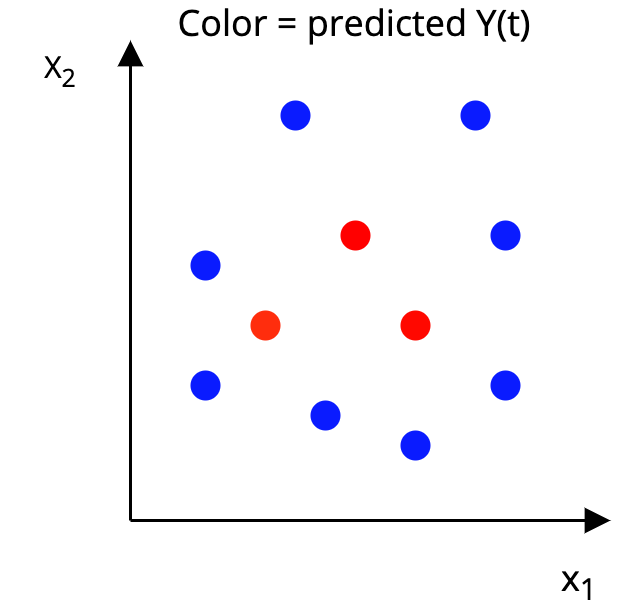### The AHB Algorithm

Step 2: Construct a p-dimensional box around each unit, such that, in the box:

 There are many units Predicted outcomes are similar Predictions from outcome averages are similar to ML predictions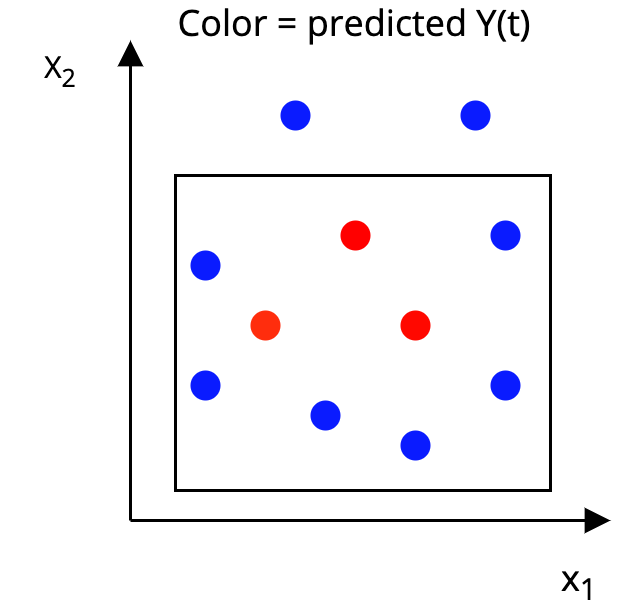### The AHB Algorithm

Step 2: Construct a p-dimensional box around each unit, such that, in the box:

 There are many units Predicted outcomes are similar Predictions from outcome averages are similar to ML predictions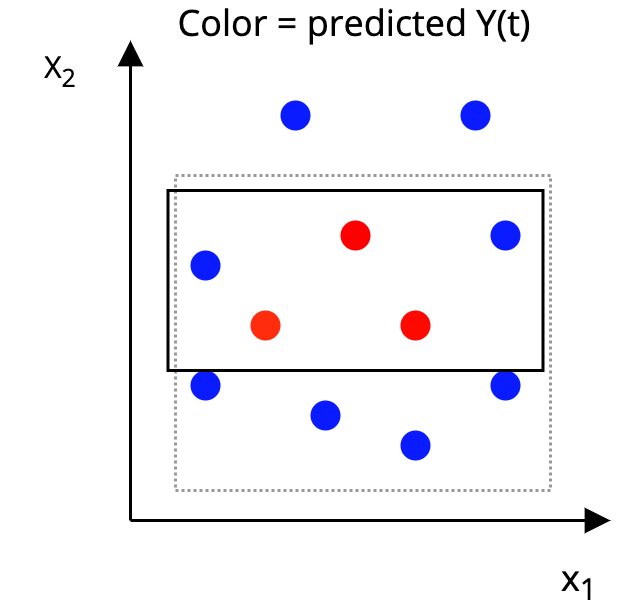### The AHB Algorithm

Step 2: Construct a p-dimensional box around each unit, such that, in the box:

 There are many units Predicted outcomes are similar Predictions from outcome averages are similar to ML predictions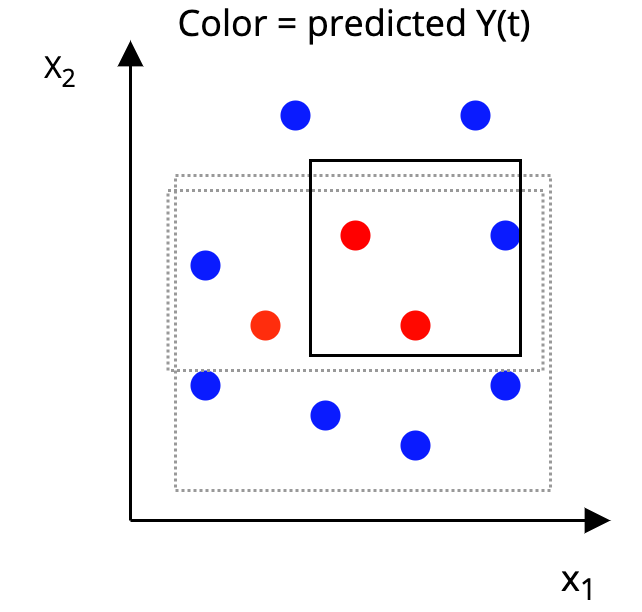### The AHB Algorithm

Step 3: Match together units that are in the same box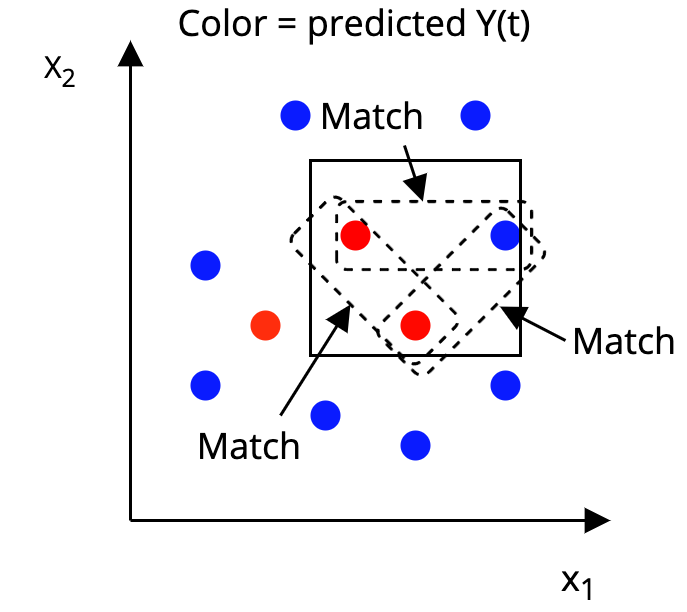That's it!

### AHB Formally

$\min_{\mathbf{H}} Err(\mathbf{H}, \mathbf{y}) + Var(\mathbf{H}, \mathbf{y}) - n(\mathbf{H})$

where:

• $\mathbf{H}$ is the hyper-box we want to find
• $Err(\mathbf{H}, \mathbf{y})$ is the outcome prediction error for all points in $\mathbf{H}$
• $Var(\mathbf{H}, \mathbf{y})$ is the outcome variance between points in $\mathbf{H}$
• $n(\mathbf{H})$ is the number of points in $\mathbf{H}$

### AHB Formally

In practice, we solve, for every unit, $i$:

$\min_{\mathbf{H}_i} \sum_{j=1}^n \sum_{t=0}^1 w_{ij}|\hat{f}(\mathbf{x}_i, t) - \hat{f}(\mathbf{x}_j, t)| - \gamma\sum_{j=1}^n w_{ij}$

• $w_ij$ denotes whether unit $j$ is contained in box $i$
• $\hat{f}(\mathbf{x}, t)$ is the predicted outcome

This function controls all terms previously introduced.

### Two solution methods for AHB

#### An exact solution via a MILP formulation

• Can be solved by any popular MIP solver (CPLEX, Gurobi, GLPK...)
• Simple preprocessing steps make solution relatively fast

### Two solution methods for AHB

#### A fast approximate algorithm

1. Determine which unit is closest in absolute distance along each covariate
2. Expand the box in the direction with the lowest predicted outcome variance
3. Repeat until a desired threshold of matches or loss is met

### AHB Performance

Test case: Lalonde data

• Effect of training program on income
• Several samples, two observational (PSID, CPS), one experimental (NSW)
• Idea: match experimental treated units (N=185) to observational controls (N>2000) to recover experimental estimates.
• Matching covariates: Age, Education, Prior income, Race, Marital status

### AHB Performance

Test case: Lalonde data

Who gets closest to experimental?

Method CPS sample PSID sample
Experimental 1794$1794$
AHB1720$1762$
Naive-7729-14797
Full Matching708816
Prognostic13192224
CEM3744-2293
Mahalanobis1181-804

### AHB Performance

 Recall: ideally, adaptive bins would look like this:AHB outputs these bins on the Lalonde data: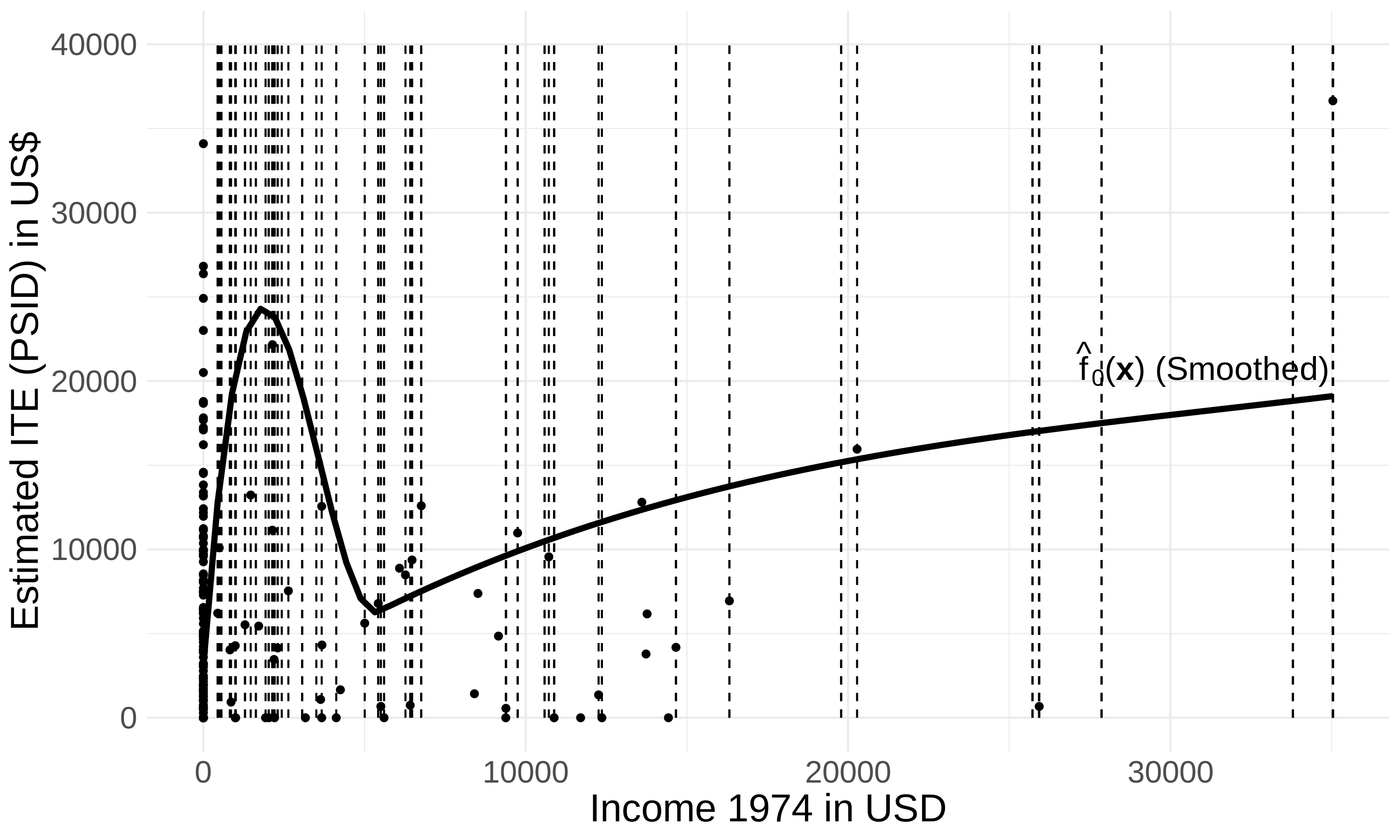Pretty close to the theoretical ideal!

### Are our Matches interpretable?

AME/AHB matches vs. Pscore matches

 Age Education Black Married HS Degree Pre income Post Income (outcome) Treated unit we want to match 22 9 no no no 0 3595.89 Matched controls: prognostic score 44 9 yes no no 0 9722 22 12 yes no yes 532 1333.44 18 10 no no no 0 1859.16 Matched controls: AHB 22 9 no no no 0 1245 20 8 no no no 0 5862.22 24 10 yes no no 0 4123.85

### Are our Matches interpretable?

AHB Interactive output

### Extensions of the AME framework

The AME framework has already been extended to...

 Instrumental Variables [Awan, Liu, Morucci, Roy, Rudin, Volfovsky (UAI 2019)]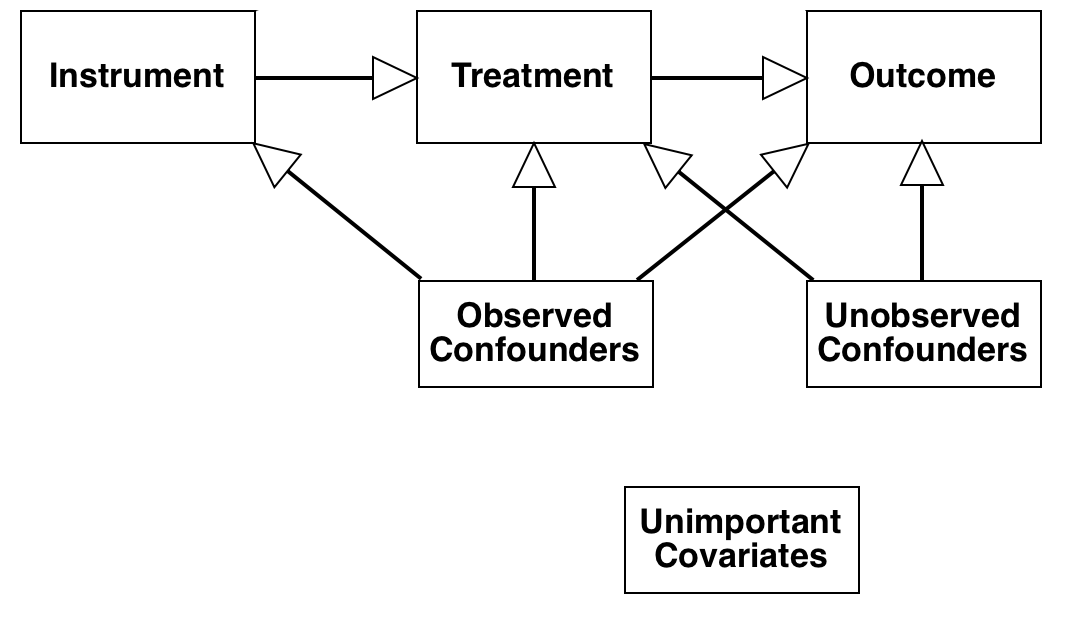Network Interference [Morucci, Orlandi, Roy, Rudin, Volfovsky (AISTATS 2020)]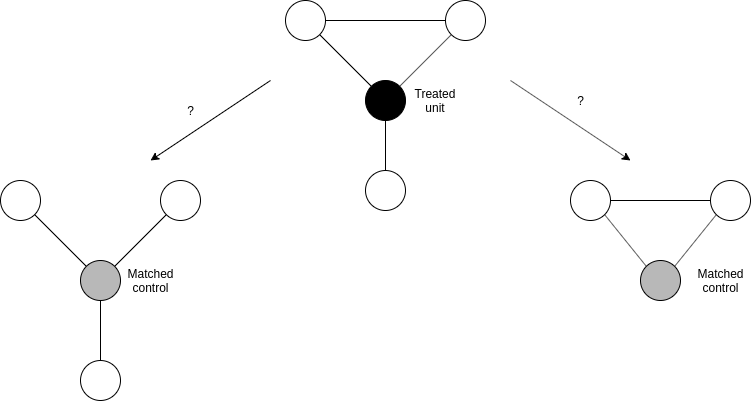### Extensions of the AME framework

...but there are many other natural avenues:

• Matching with non-standard data such as text or images
• Matching with spatio/temporal data
• AME for difference-in-differences estimation
• ...and more!

Currently we're working on:

• Constructing estimators for matched groups that are asymptotically well-behaved
• Using matching to facilitate sensitivity analysis in massive observational datasets

### Extensions of the AME framework

...but there are many other natural avenues:

• Matching with non-standard data such as text or images
• Matching with spatio/temporal data
• AME for difference-in-differences estimation
• ...and more!

Currently we're working on:

• Constructing estimators for matched groups that are asymptotically well-behaved
• Using matching to facilitate sensitivity analysis in massive observational datasets

### Software

https://almost-matching-exactly.github.io/

### Conclusion

Interpretability of causal estimates is important!

• We propose matching methods that produce interpretable and accurate CATE estimates
• Our methods take advantage of machine learning backends to boost accuracy
• Our methods explain both estimates in terms of cases, and why cases were selected in terms of covariate similarity.